# Algebra 1 Chapter 5 Test Answer Key

We thoroughly check each answer to a question to provide you with the most correct answers. Found a mistake? Let us know about it through the REPORT button at the bottom of the page.

1 Solve and graph the inequality: k – 7 < 5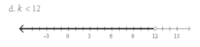2. Solve and graph the inequality: -2 ≥ w – 6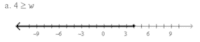3. Solve the inequality: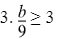• b ≥ 27
• b ≥ 12
• b ≥ -27

4. Solve the inequality: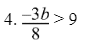• b < -24
• b > -24
• b > 6

5. Solve the inequality: -5f < 25

• f > -125
• f > -5
• f < -5

6. Solve the inequality:

5a + 9 – 7a > 13

• a > -2
• a < -2
• a > 1

7. Solve in the inequality: 8h + 4 > 68

• h < 8
• h > 8
• h > 64

8. Solve the inequality:

-18(2z + 8) < -6(6z – 5)

• Empty set
• z > 17
• z < 174
• All real numbers

9. Solve the inequality:

5n – 3(n – 6) ≥ 0

• n ≥ 9
• n ≥ -9
• n ≤ 9

10. Solve the inequality:

-4 < 3t + 5 ≤ 20

• t < -3
• t < -3 or t ≥ 5
• -3 < t ≤ 5

11. Solve the inequality: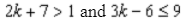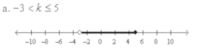12. Which is the graph of the inequality: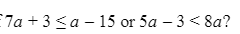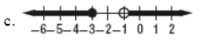13. Graph the inequality:

3y > 2x – 3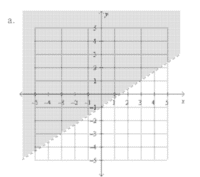14. Juan’s income y consists of at least \$37,500 salary plus 5% commission on all of his sales x. Which inequality represents Juan’s income in one year?

• y ≥ 37,500 + 0.05x
• y ≤ 37,500 + 5x
• y ≥ 37,500 + 5

15. Which inequality has the solution set shown in the graph?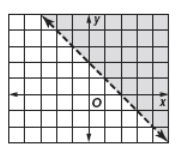• y > -x + 2
• y > -x + 1
• y < -x + 1

## Chapter 5 Test Algebra 1 Test Answers

rate of change
slope
slope
m=rise over run
can be fractions
rise
y-axis
run
x-axis
delta
change in…
horizontal line
m=0
vertical line
m=undefined(# divided by 0)
direct variation formula
y=kx
y is…
dependent
x is…
independent
slope intercept form
y=mx+b
point slope form
y-y=m(x-x)
y=mx+b
m=slope,
b=y-intercept
point slope(intercept)
when graphing start on y-axis
standard form
Ax+Bx=C
least useful
multiply or divide=like terms include variable
add or subtract=like terms do not include the variable
A, B, and C
are integers
graphing standard form
graph as points not a line